# Medium Transmission Line: What is it? (Plus ABCD Parameters)

## What is Medium Transmission Line?

A medium transmission line is defined as a transmission line with an effective length more than 80 km (50 miles) but less than 250 km (150 miles). Unlike a short transmission line, the line charging current of a medium transmission line is appreciable and hence the shunt capacitance must be considered (this is also the case for long transmission lines). This shunt capacitance is captured within the admittance (“Y”) of the ABCD circuit parameters.

The ABCD parameters of a medium length transmission line is calculated using a lumped shunt admittance, along with the lumped impedance in series to the circuit. These lumped parameters of a medium length transmission line can be represented using three different models, namely:

1. Nominal Π representation (nominal pi model)
2. Nominal T representation (nominal T model)
3. End Condenser Method

Let’s now go into the detailed discussion of these above-mentioned models, deriving the ABCD parameters for medium transmission lines.

### Nominal Π Representation of a Medium Transmission Line

In case of a nominal Π representation (i.e. nominal pi model), the lumped series impedance is placed at the middle of the circuit whereas the shunt admittances are at the ends. As we can see from the diagram of the Π network below, the total lumped shunt admittance is divided into 2 equal halves, and each half with value Y ⁄ 2 is placed at both the sending and the receiving end while the entire circuit impedance is between the two.

The shape of the circuit so formed resembles that of a symbol Π, and for this reason, it is known as the nominal Π representation of a medium transmission line. It is mainly used for determining the general circuit parameters and performing load flow analysis.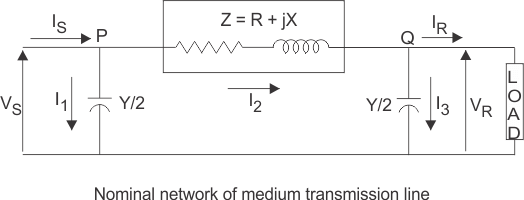As we can see here, VS and VR is the supply and receiving end voltages respectively, and Is is the current flowing through the supply end. IR is the current flowing through the receiving end of the circuit. I1 and I3 are the values of currents flowing through the admittances. And I2 is the current through the impedance Z

Now applying KCL, at node P, we get.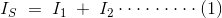Similarly applying KCL, to node Q.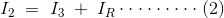Now substituting equation (2) to equation (1)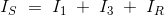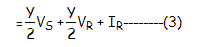Now by applying KVL to the circuit,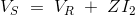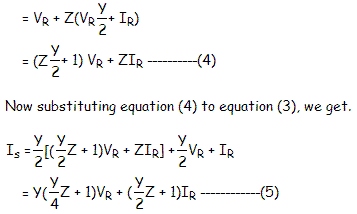Comparing equation (4) and (5) with the standard ABCD parameter equations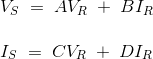We derive the ABCD parameters of a medium transmission line as: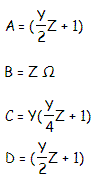### Nominal T Representation of a Medium Transmission Line

In the nominal T model of a medium transmission line the lumped shunt admittance is placed in the middle, while the net series impedance is divided into two equal halves and placed on either side of the shunt admittance. The circuit so formed resembles the symbol of a capital T, and hence is known as the nominal T network of a medium length transmission line and is shown in the diagram below.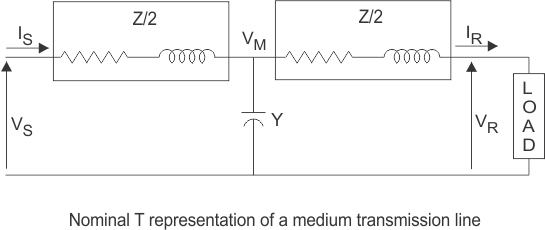Here also Vs and Vr is the supply and receiving end voltages respectively, and
Is is the current flowing through the supply end.
Ir is the current flowing through the receiving end of the circuit.
Let M be a node at the midpoint of the circuit, and the drop at M, be given by Vm.
Applying KVL to the above network we get,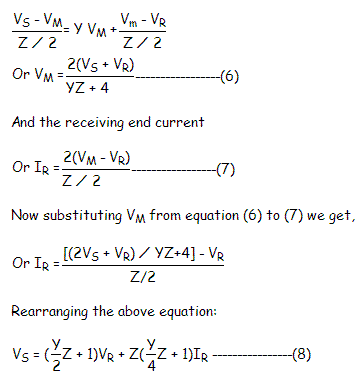Now the sending end current is,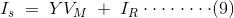Substituting the value of VM to equation (9) we get,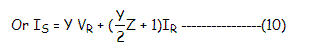Again comparing equation (8) and (10) with the standard ABCD parameter equations,The parameters of the T network of a medium transmission line are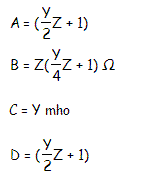### End Condenser Method

In this method, the capacitance of the line is limped or concentrated at the receiving or load end. This method of localizing the line capacitance at the load end overestimates the effects of capacitance.

Want To Learn Faster? 🎓
Get electrical articles delivered to your inbox every week.
No credit card required—it’s 100% free.